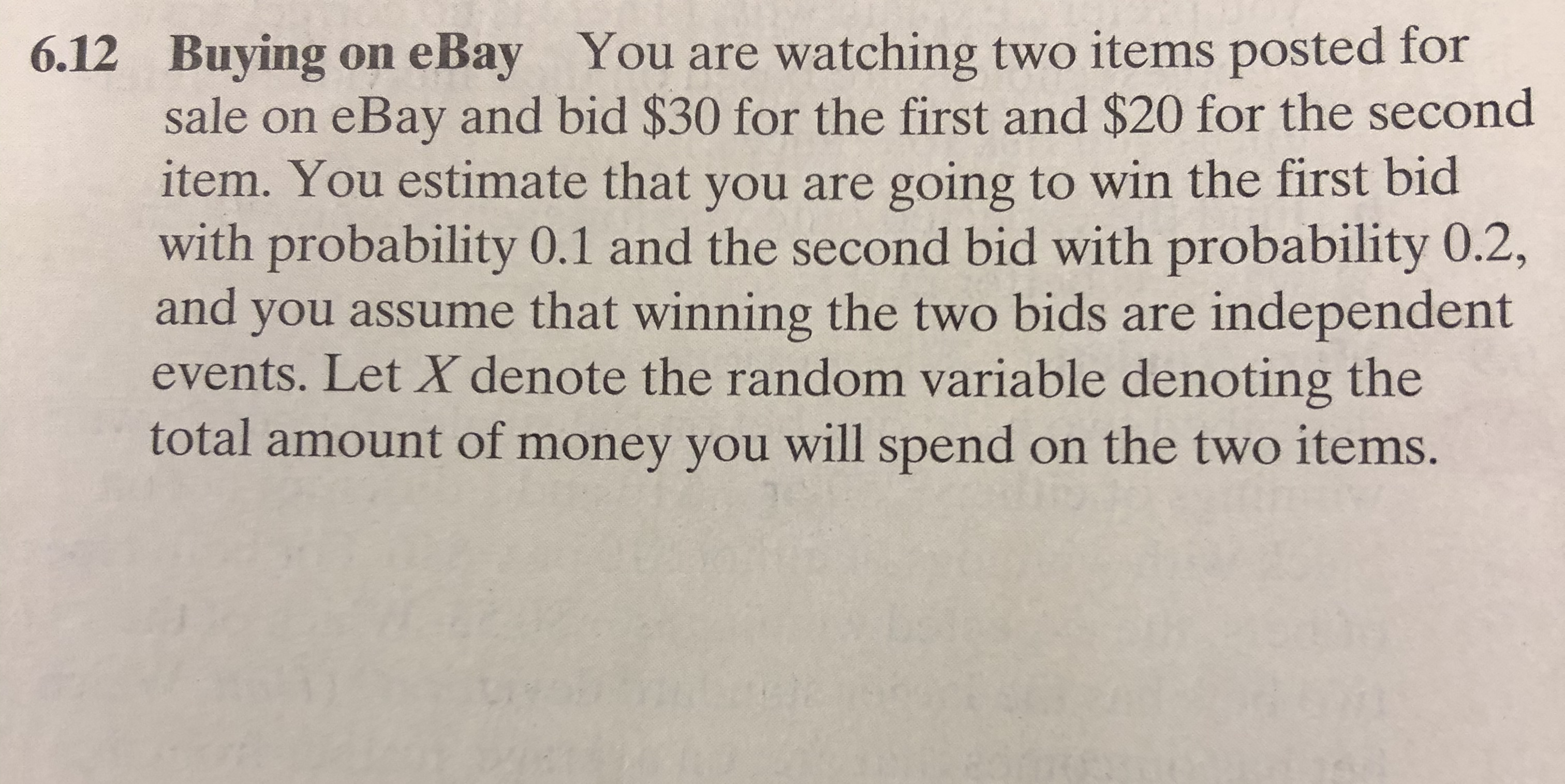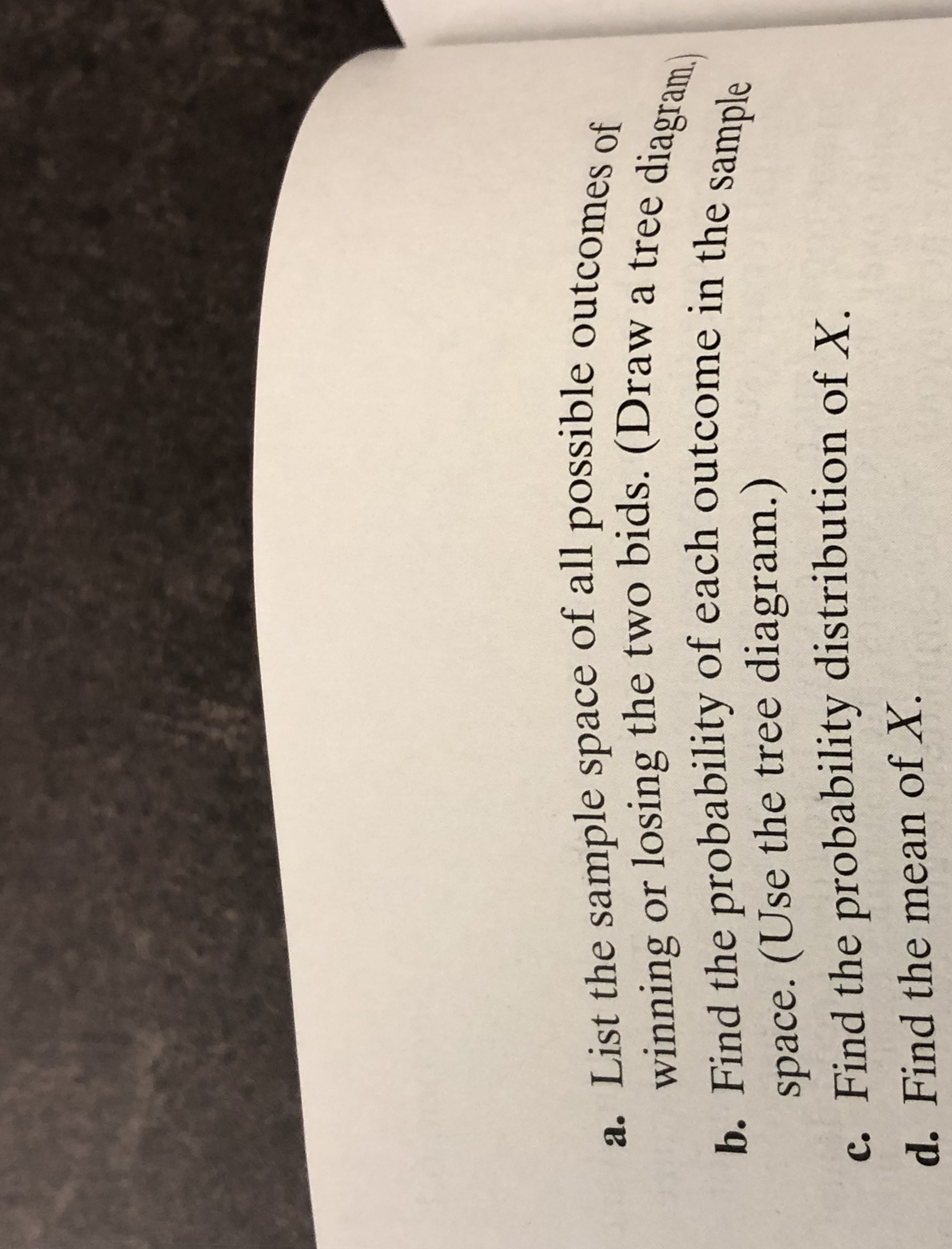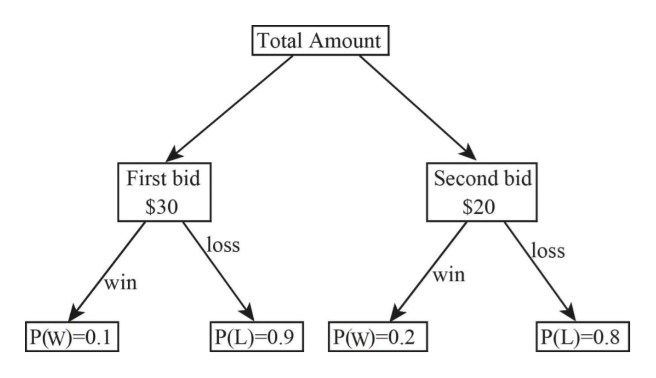# 6.12 Buying on eBay You are watching two items posted forsale on eBay and bid \$30 for the first and \$20 for the seconditem. You estimate that you are going to win the first bidwith probability 0.1 and the second bid with probability 0.2,and you assume that winning the two bids are independentevents. Let X denote the random variable denoting thetotal amount of money you will spend on the two items. a. List the sample space of all possible outcomes ofwinning or losing the two bids. (Draw a tree diagram.)b. Find the probability of each outcome in the samplespace. (Use the tree diagram.)c. Find the probability distribution of X.d. Find the mean of X.

Question
78 viewshelp_outlineImage Transcriptionclose6.12 Buying on eBay You are watching two items posted for sale on eBay and bid \$30 for the first and \$20 for the second item. You estimate that you are going to win the first bid with probability 0.1 and the second bid with probability 0.2, and you assume that winning the two bids are independent events. Let X denote the random variable denoting the total amount of money you will spend on the two items. fullscreenhelp_outlineImage Transcriptionclosea. List the sample space of all possible outcomes of winning or losing the two bids. (Draw a tree diagram.) b. Find the probability of each outcome in the sample space. (Use the tree diagram.) c. Find the probability distribution of X. d. Find the mean of X. fullscreen
check_circle

Step 1

Hey there! Thank you for posting the question. Since your question has more than 3 parts, we are solving the first 3 parts for you, according to our policy. If you need help with any of the other parts, please re-post the question and mention the part you want answered.

Step 2

a)

The first outcome in sample space is WW, here first W represents the winning of first bid and second W represents the winning of second bid. Other outcomes are WL, LW, and LL. In WL the first W represents the winning of first bid and second L represents the loosing of second bid and in LW in that first L represents the loosing of first bid and second W represents the winning of second bid and LL in that first L represents the loosing of first bid and second L represents the loosing of second bid.

Thus, the possible sample space is {WW, WL, LW, LL}.

Tree diagram:help_outlineImage TranscriptioncloseTotal Amount Second bid First bid \$30 \$20 loss loss win win P(W)-0.1 P(L) 0.9 P(W)-0.2 P(L)=0.8 fullscreen
Step 3

b)

The outcomes of the sample space is {WW, WL, LW, LL}.

Here, the probability of winning of first bid is 0.1 and winning of second bi...

### Want to see the full answer?

See Solution

#### Want to see this answer and more?

Solutions are written by subject experts who are available 24/7. Questions are typically answered within 1 hour.*

See Solution
*Response times may vary by subject and question.
Tagged in

### Data